Chapter 4: Inequalities

# 4.3 Linear Absolute Value Inequalities

Absolute values are positive magnitudes, which means that they represent the positive value of any number.

For instance, | −5 | and | +5 | are the same, with both having the same value of 5, and | −99 | and | +99 | both share the same value of 99.

When used in inequalities, absolute values become a boundary limit to a number.

Example 4.3.1

Consider $| x | < 4.$ This means that the unknown $x$ value is less than 4, so $| x | < 4$ becomes $x < 4.$ However, there is more to this with regards to negative values for $x.$ | −1 | is a value that is a solution, since 1 < 4. However, | −5 | < 4 is not a solution, since 5 > 4. The boundary of $| x | < 4$ works out to be between −4 and +4. This means that $| x | < 4$ ends up being bounded as $-4 < x < 4.$ If the inequality is written as $| x | \le 4$, then little changes, except that $x$ can then equal −4 and +4, rather than having to be larger or smaller. This means that $| x | \le 4$ ends up being bounded as $-4 \le x \le 4.$

Example 4.3.2

Consider $|x|$ > $4.$

This means that the unknown $x$ value is greater than 4, so $|x|$ > $4$ becomes $x$ > $4.$ However, the negative values for $x$ must still be considered.

The boundary of $|x|$ > $4$ works out to be smaller than −4 and larger than +4.

This means that $|x|$ > $4$ ends up being bounded as $x < -4 \text{ or } 4 < x.$ If the inequality is written as $| x | \ge 4,$ then little changes, except that $x$ can then equal −4 and +4, rather than having to be larger or smaller. This means that $|x| \ge 4$ ends up being bounded as $x \le -4 \text{ or } 4 \le x.$

When drawing the boundaries for inequalities on a number line graph, use the following conventions:

For ≤ or ≥, use [brackets] as boundary limits.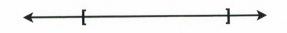For < or >, use (parentheses) as boundary limits.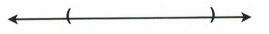Equation Number Line
$| x | <4$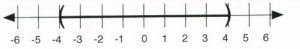$| x | \le 4$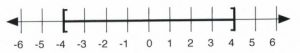$| x |$ > $4$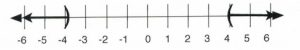$| x | \ge 4$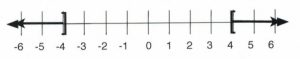When an inequality has an absolute value, isolate the absolute value first in order to graph a solution and/or write it in interval notation. The following examples will illustrate isolating and solving an inequality with an absolute value.

Example 4.3.3

Solve, graph, and give interval notation for the inequality $-4 - 3 | x | \ge -16.$

First, isolate the inequality:

$\begin{array}{rrrrrl} -4&-&3|x|& \ge & -16 &\\ +4&&&&+4& \text{add 4 to both sides}\\ \hline &&\dfrac{-3|x|}{-3}& \ge & \dfrac{-12}{-3}&\text{divide by }-3 \text{ and flip the sense} \\ \\ &&|x|&\le & 4 && \end{array}$

At this point, it is known that the inequality is bounded by 4. Specifically, it is between −4 and 4.

This means that $-4 \le | x | \le 4.$

This solution on a number line looks like: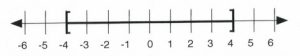To write the solution in interval notation, use the symbols and numbers on the number line: $[-4, 4].$

Other examples of absolute value inequalities result in an algebraic expression that is bounded by an inequality.

Example 4.3.4

Solve, graph, and give interval notation for the inequality $| 2x - 4 | \le 6.$

This means that the inequality to solve is $-6\le 2x - 4\le 6$:

$\begin{array}{rrrcrrr} -6&\le & 2x&-&4&\le & 6 \\ +4&&&+&4&&+4 \\ \hline \dfrac{-2}{2}&\le &&\dfrac{2x}{2}&&\le & \dfrac{10}{2} \\ \\ -1 &\le &&x&&\le & 5 \end{array}$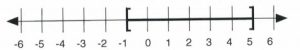To write the solution in interval notation, use the symbols and numbers on the number line: $[-1,5].$

Example 4.3.5

Solve, graph, and give interval notation for the inequality $9 - 2 | 4x + 1 |$ > $3.$

First, isolate the inequality by subtracting 9 from both sides:

$\begin{array}{rrrrrrr}9&-&2|4x&+&1&>&3 \\ -9&&&&&&-9 \\ \hline &&-2|4x&+&1|&>&-6 \end{array}$

Divide both sides by −2 and flip the sense:

$\begin{array}{rcc}\dfrac{-2|4x+1|}{-2}&>&\dfrac{-6}{-2} \\ |4x+1|&<&3 \end{array}$

At this point, it is known that the inequality expression is between −3 and 3, so $-3 < 4x + 1 < 3.$ All that is left is to isolate $x$. First, subtract 1 from all three parts:

$\begin{array}{rrrrrrr} -3&<&4x&+&1&<&3 \\ -1&&&-&1&&-1 \\ \hline -4&<&&4x&&<&2 \\ \end{array}$

Then, divide all three parts by 4:

$\begin{array}{rrrrr} \dfrac{-4}{4}&<&\dfrac{4x}{4}&<&\dfrac{2}{4} \\ \\ -1&<&x&<&\dfrac{1}{2} \\ \end{array}$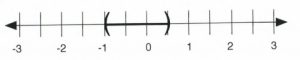In interval notation, this is written as $\left(-1,\dfrac{1}{2}\right).$

It is important to remember when solving these equations that the absolute value is always positive. If given an absolute value that is less than a negative number, there will be no solution because absolute value will always be positive, i.e., greater than a negative. Similarly, if absolute value is greater than a negative, the answer will be all real numbers.

This means that:

$\begin{array}{c}| 2x - 4| < -6 \text{ has no possible solution } (x \ne \mathbb{R}) \\ \\ \text{and}\\ \\ |2x-4| > -6 \text{ has every number as a solution and is written as } (-\infty, \infty) \end{array}$

Note: since infinity can never be reached, use parentheses instead of brackets when writing infinity (positive or negative) in interval notation.

# Questions

For questions 1 to 33, solve each inequality, graph its solution, and give interval notation.

1. $| x | < 3$
2. $| x | \le 8$
3. $| 2x | < 6$
4. $| x + 3 | < 4$
5. $| x - 2 | < 6$
6. $| x - 8 | < 12$
7. $| x - 7 | < 3$
8. $| x + 3 | \le 4$
9. $| 3x - 2 | < 9$
10. $| 2x + 5 | < 9$
11. $1 + 2 | x - 1 | \le 9$
12. $10 - 3 | x - 2 | \ge 4$
13. $6 - | 2x - 5 |$ > $3$
14. $| x |$ > $5$
15. $| 3x |$ > $5$
16. $| x - 4 |$ > $5$
17. $| x + 3 |$ > $3$
18. $| 2x - 4 |$ > $6$
19. $| x - 5 |$ > $3$
20. $3 - | 2 - x | < 1$
21. $4 + 3 | x - 1 | < 10$
22. $3 - 2 | 3x - 1 | \ge -7$
23. $3 - 2 | x - 5 | \le -15$
24. $4 - 6 | -6 - 3x | \le -5$
25. $-2 - 3 | 4 - 2x | \ge -8$
26. $-3 - 2 | 4x - 5 | \ge 1$
27. $4 - 5 | -2x - 7 | < -1$
28. $-2 + 3 | 5 - x | \le 4$
29. $3 - 2 | 4x - 5 | \ge 1$
30. $-2 - 3 | - 3x - 5| \ge -5$
31. $-5 - 2 | 3x - 6 | < -8$
32. $6 - 3 | 1 - 4x | < -3$
33. $4 - 4 | -2x + 6 |$ > $-4$